# 1. Introduction to MSTs

Given: undirected connecte graph `G` with positive edge weights.
def. Spanning tree `T`
is a subgraph of `G`, that is both tree (connected, acyclic) and spanning(all vertices are included).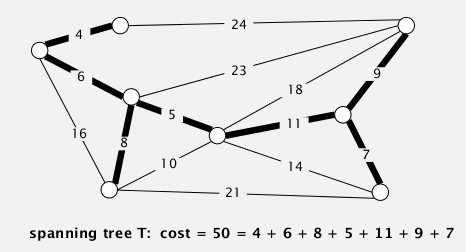⇒ Goal: find a spanning tree with minimum weight sum.

# 2. Greedy Algorithm

assumptions for simplification:

• edge weights are distinct
• graph is connected

→ MST uniquely exists.

## cut property

def. a cut of a graph is a partition of its vertices into 2 non-empty sets.
def. a crossing-edge (wrt a cut) is an edge connecting vertex from one set to another.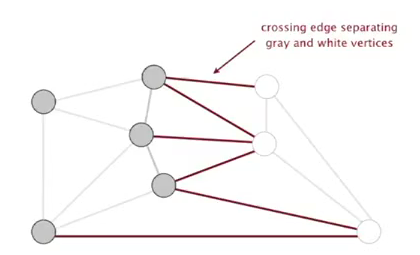prop. Given any cut, the crossing edges with minimum weight is in the MST.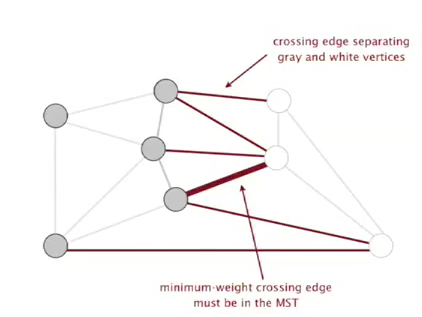proof.
Given a cut. {S1,S2} are the two set of vertices, let `e` be the min-weighted edge among all crossing-edges.

If `e` is not in the MST
→ exist another crossing-edge, `f`, in the MST (otherwise not connected)
→ adding `e` to the MST will create a cycle (tree property)
→ the edge `f` will be in this cycle
→ removing `f` and adding `e` will give us another spanning tree (!)
→ this new spanning tree has smaller weight sum ⇒ contradiction, CQFD.

## Greedy MST algo

[algo ] Greedy MST

• initialize: all edges not selected (colored gray)
• find any cut with all crossing-edge gray
• use this cut and select the min-weighted crossing edge (color the edge as black)
• repeat V-1 times.

prop. the greedy algorithm gets the MST.
pf.

• any selected (black) edges are in the MST (according to the cut property)
• If we haven't selected V-1 edges → there is always a cut with all crossing-edges gray. (证明algo不会卡死)

(if edge weight not distinct, the proof fails, but can be fixed)

efficient implementations:

• how to choose the cut each time?
• how to find min-weighted crossing-edge?

⇒ Kruskal & Prim

# 3. Edge-Weighted Graph API

## Edge API

Edge abstraction: make Edge comparable.

```public class Edge implements Comparable<Edge>{
Edge(int v, int w, double weight);
int either();// get one of the endpoint of edge (as we are in undirected graph contex here)
int other(int v);// get the other endpoint
int compareTo(Edge that);// compare by edge weight
double weight();
}
```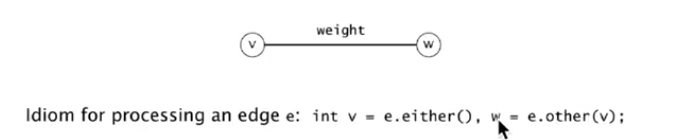## Edge-weighted Graph API

adj-list implementation: `Bag<Edge>[] adj;`(for undirected graph, each edge appears twice in adj)

```public class EdgeWeightedGraph{
private final int V;
EdgeWeightedGraph(int V){
this.V = V;
for(int v=0;v<V;v++) adj[v] = new Bag<Edge>();
}
void addEdge(Edge e){// use the Edge class instead of directly v and w
int v = e.either(), w = e.other();
}
Iterable<Edge> adj(int v){//get Edges incident to v
}
Iterable<Edge> edges();// get all Edges
}
```

(allow self-loops and parallel edges)

## MST API

```public class MST{
MST(EdgeWeightedGraph G);//compute the MST
Iterable<Edge> edges();// selected edges in the MST
double weight();// sum of all edge weights in MST
}
```

# 4. Kruskal's Algorithm

[algo]

• consider edges in ascending order of weight,
• add the edge to MST unless it creates a cycle.

In the running of Kruskal: we have several small connect components and they merge with each other until we get MST.

## correctness

prop. Kruskal's algo works.
pf
(idea: proove that Kruskal is a special case of the greedy algorithm, ie. how to select the specific cut)
suppose Kruskal's algo selects(colored black) an edge `e=v-w`
→ select a cut = vertices connected to v in the (constructing) MST; and the rest vertices.
→ for this cut, there is no black crossing edges
→ moreover among all crossing edges of the cut the edge e has the smallest weight!! (by def of Kruskal) CQFD

## implementation

• how to test if adding an edge will create a cycle ?

DFS from v to w? → O(V)
Union-Find ! O(lg*V) ☺ (almost constant time)

if `find(v)==find(w)`, then we know adding e will create a cycle.

• considering edges in order? → use a prority queue.
```public class KruskalMST extends MST{
private Bag<Edge> mst = new Bag<Edge>();
public KruskalMST(EdgeWeightedGraph G){
MinPQ<Edge> pq = new MinPQ<Edge>();
// build pq --> can be optimized to O(n) if build bottom-up
for(Edge e: G.edges()) pq.insert(e);
UF uf = new UF(G.V());// build a UF of V elements
while(!pq.isEmpty() && mst.size()<G.V()-1){
Edge e = pq.delMin();
int v = e.either(),w=e.other(v);
if( uf.connecte(v,w) ) continue;
uf.union(v,w);
}
}
public Iterable<Edge> edges(){
return this.mst;
}
}
```

## complexity

running time: O(ElogE)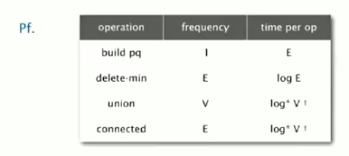# 5. Prim's Algorithm

since 1930...
Idea: start from a vertex and grows the tree `T` to MST.

[algo]

• Add to the tree T the edge that have exactely one endpoint in T and with minimum weight,
• repeat V-1 times.

In the running of Prim: there is always ONE connnected component .

## Correctness

prop. Prim's algo works.
pf.
suppose edge e is the min-weighted edge connect a vertex in T with a vertex out of T.
→ select the cut = vertices in the tree T; vertices out of T
→ by def, there is no black crossing edge
→ e is the min-weighed edge by def of Prim. CQFD

## implementation

challenge: how to find such an edge (connect T and other vertex, with min weight) ?
⇒ priority queue

### "lazy" implementation

[algo]

• Maintain a PQ of edges that connect T and the rest vertices.
• `e = pq.delMin(), e = v-w`, if `v` and `w` are both in T (as edges in pq might become obsolete as T grows) ⇒ just disregard it
• to maintain the pq: add all incident edges(with other endpoint not in T) of the newly added vertex to pq
```public class LazyPrimMST{
private Bag<Edge> mst;
LazyPrimMST(EdgeWeightedGraph G){
boolean[] marked = new boolean[G.V()]; // vertices in T
MinPQ<Edge> pq = new MinPQ<Edge>();
this.mst = new Bag<Edge>();
marked = 0; // add vertex 0 to T
while(!pq.isEmpty() && this.mst.size()<G.V()-1){
e = pq.delMin();
int v = e.either(), w = e.other(v);
if(marked[v] && marked[w]) continue;//ignore obsolete edges
v = marked[v] ? w : v;// v is the newly added vertex
marked[v] = true;
if(!marked[e.other(v)])
pq.insert(e);
}
}
}
}
```

Running time: O(ElgE)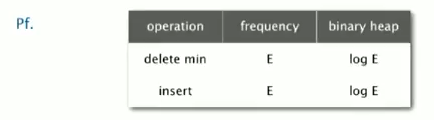space: O(E) in worst time.

### "eager" implementation

Idea:

use a PQ of vertices, priority of vertex v := min-weight of edge that connects v to T.

[algo]

• Get from pq the vertex `v` that is closest to T, add it to T.
Update pq -- consider `v`'s incident edge `e=v-w:`
• if w in T → ignore
• else:
• if w in pq → add w to pq
• else → if `v-w` has smaller weight than the current priority, update `w`'s priority.
• repeat till get V-1 edges.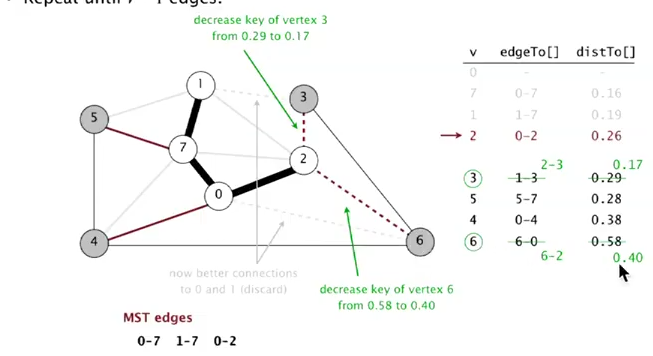key implementation component: a MinPQ that supports priority(key) update.

```class IndexMinPQ<Key extends Comparable<Key>>{
IndexMinPQ(int N);// indices of elements: 0...N-1
void insert(int i, Key key);
void decreaseKey(int i, Key key);// update the key(priority) of element-i
int delMin();
int size();
}
```

implementation of such a PQ:

Use same code as standart PQ (maintain a heap[] array).
Elements are always accessed by "index", in range 0...N-1. maintain 3 parallel arrays: `keys[], pq[], qp[]`:

• `keys[i]`: is the priority of element i (the element with index=i)
• `pq[i]`: is the index of the element in the heap position i (ie. in heap[i] is pq[i]th element )
• `qp[i]`: is heap position of element i ( ⇔ the ith element is in heap[qp[i]] )

to `decreaseKey(i,key)`: change `keys[i]`, then call `siftup(qp[i])`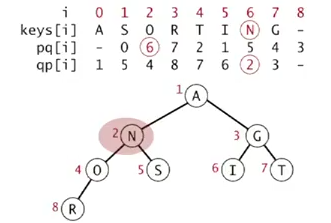summery of pq implementations: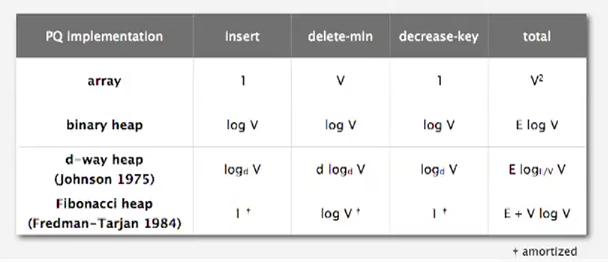# 6. MST Context

• unsolved pb: does a linear MST algo exists?

(recap: for UF, tarjan has prooved that linear algo doesn't exist — although Nlg*N is fast enough...)
@_@...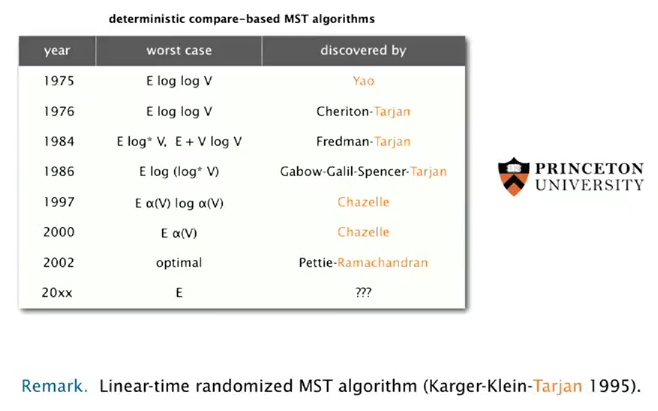(这个Yao是清华那个Yao吧?)

• Euclidean MST

Given N points in plane, edge weight := Euclidean distance. (dense graph, E = V2)
→ exploit geomerty, O(NlgN)

• clustering

k-clustering (~ dist-fcn)
single-link clustering (def. dist of clusters = dist of 2 closest elements in each cluster)
→ Kruskal...